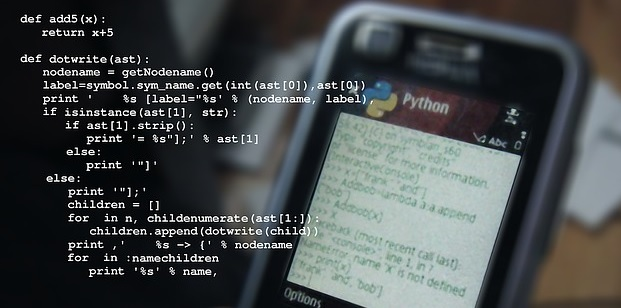### What is Python?

Python is a high-level easy use computer programming language.It is the most powerful object-oriented, high-level computer programming language for making any programme by Guido van Rossum.

It is a general-purpose computer programming language. It has a wide range of applications such as Web development, Mathematical and Graphics User Interface.

The language Python is very easy to learn and understandable among all computer languages.

Its syntax is very simple to write and read the programming coding.

• IBM
• NetFlix
• Firefox
• NASA
• DropBox

### Features of the Python

• Free where and shareware
• Object-Oriented
• Easy to learn and understand
• It is easy to portable.
• Executable in any Operating System
• High-Level Interpreted Language
• Large Standard Libraries
• Codes length are shorts

### Data Types and Variables

Integers (Numbers)

x = (23)

Float (Decimals)

x=(34.56)

Strings

m =(“Rana Mehtab Alam”)

Multi Variables

m, n = (8, 9)

### Commands in Python

abs (Get output in absolute value)

ceil (round up the numeric value as 9.6 = 10)

cos (cosine in radians)

floor (round down the numeric value)

log (logarithm, base e)

log10 (logarithm, base 10)

sqrt (find the square root of a numeric value)

round (round up the decimal values to the nearest as 1.2 = 1 and 1.9 = 2)

sin (sine, in radians)

max (find the maximum number from numbers list)

min (find the minimum number from numbers list)

### Rules of typing commands and defining variables.

Python is a case sensitive software so we type commands and use variables in lowercase.

There is a list of reserved words in Python that we can’t use in the variables.

• and
• assert
• break
• class
• continue
• def
• del
• elif
• else
• except
• exec
• finally
• for
• from
• global
• if
• import
• in
• is
• lambda
• not
• or
• pass
• print
• raise
• return
• try
• while

Constant

e = 2.7182818…..

pi = 3.1415926….

### How to change the line in python?

Whitespaces

We use \ to go to the next line in python

#This is a comment line

### Typing coding in the Python

print (“Rana Mehtab Alam Lunar Computer College”)

OutPut

Rana Mehtab Alam Lunar Computer College

a = (“Rana”)

print (a)

OutPut

Rana

### How to type the first code and save it in python?

print (“Welcome to the first code for python”)

to save in python it is compulsory to type .py at the end of the file name suppose if you want to type file name abc then you will type abc.py